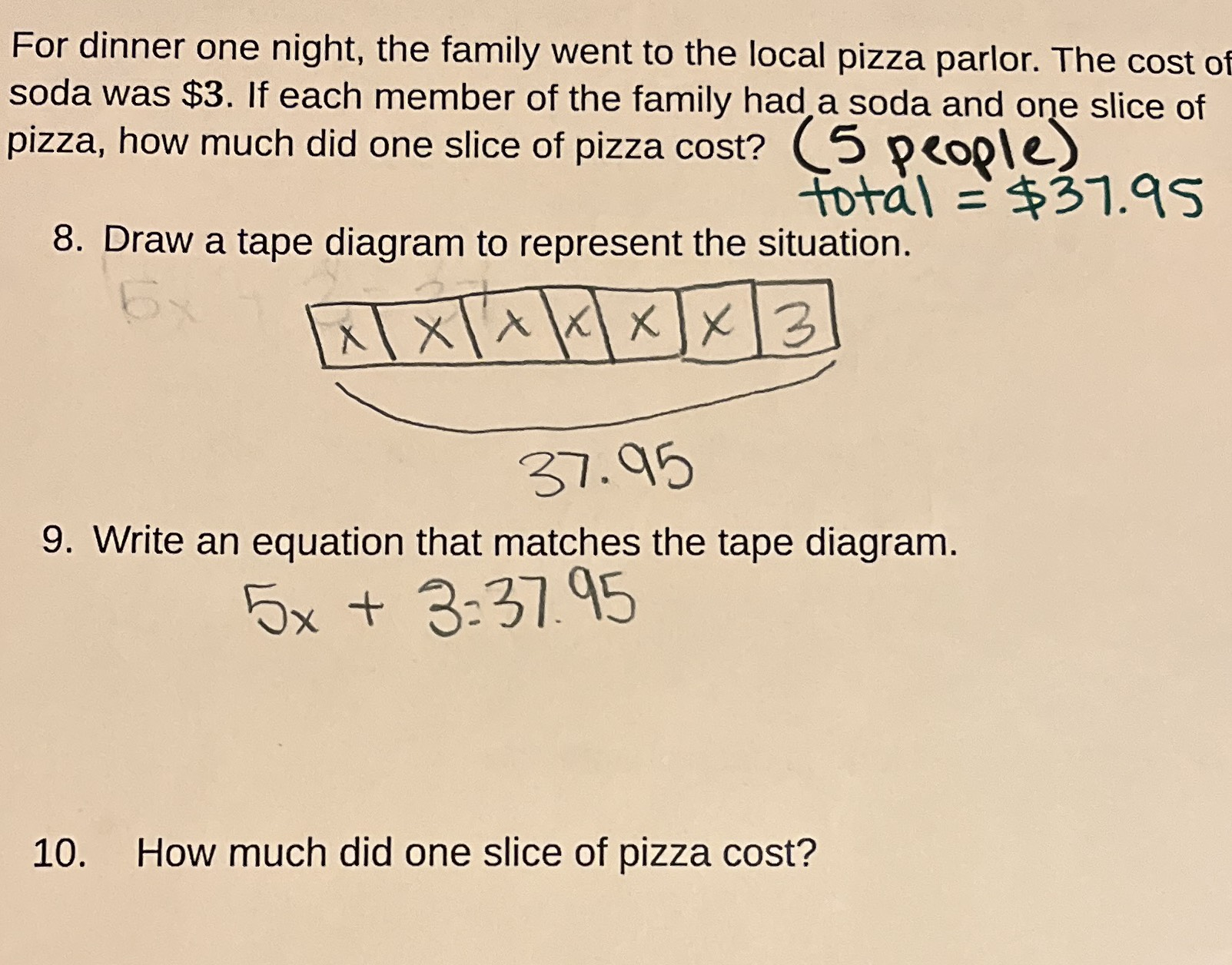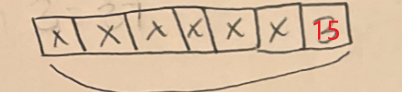### Still have math questions?

Algebra
QuestionFor dinner one night, the family went to the local pizza parlor. The cost of soda was $$\ 3$$ . If each member of the family had a soda and one slice of pizza, how much did one slice of pizza cost? (5 people)

8. Draw a tape diagram to represent the situation.

9. Write an equation that matches the tape diagram.

10. How much did one slice of pizza cost?

8.